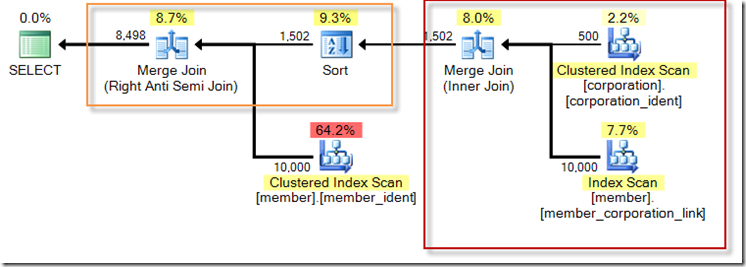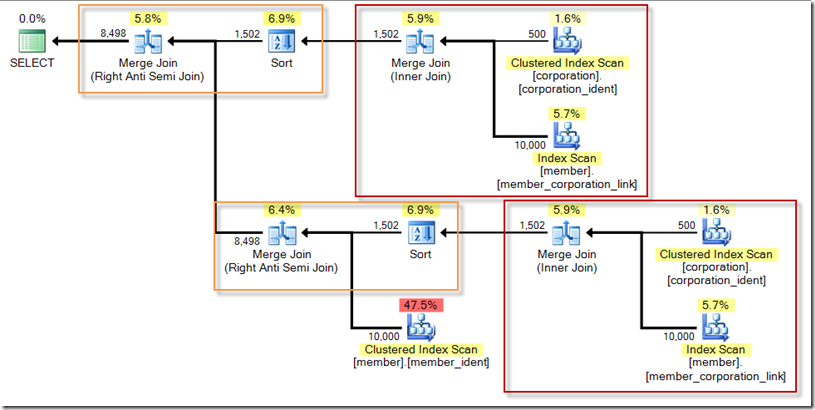Consider the following “basic_member” view definition from the Credit database:

```CREATE VIEW [dbo].[basic_member]
AS
SELECT  [member].[member_no],
[member].[lastname],
[member].[firstname],
[member].[middleinitial],
[member].[street],
[member].[city],
[member].[state_prov],
[member].[mail_code],
[member].[phone_no],
[member].[region_no],
[member].[expr_dt],
[member].[member_code]
FROM    [dbo].[member]
WHERE   [member].[member_no] NOT IN (SELECT    [corp_member].[member_no]
FROM      [dbo].[corp_member]);

GO
```

A simple SELECT from this view returns 8,498 rows and has the following plan shape (and I’m boxing in an “areas of interest” via SQL Sentry Plan Explorer’s rendering of the plan):We see that the view has a predicate on member_no NOT IN the corp_member table.  But what happens if the original report writer doesn’t look at the view definition and decides they need this same predicate applied at the the view reference scope (not realizing this was already taken care of)?  For example:

```SELECT  [basic_member].[member_no],
[basic_member].[lastname],
[basic_member].[firstname],
[basic_member].[middleinitial],
[basic_member].[street],
[basic_member].[city],
[basic_member].[state_prov],
[basic_member].[mail_code],
[basic_member].[phone_no],
[basic_member].[region_no],
[basic_member].[expr_dt],
[basic_member].[member_code]
FROM    [dbo].[basic_member]
WHERE   [basic_member].[member_no] NOT IN
(SELECT   [corp_member].[member_no]
FROM     [dbo].[corp_member]);
```

Like the previous query against the view, we see 8,498 rows.  But unlike the previous query, we see the following plan:Notice the redundancy – even though the result set is identical between the two versions.  And the tables I’m using are small, but you can still see the difference in scan count and logical reads.

Query Against View